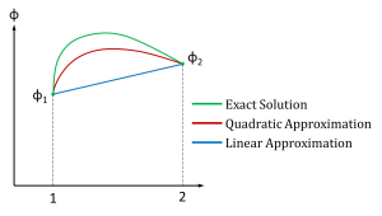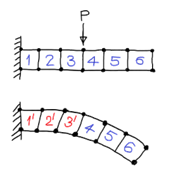## Posts

### Photoresistors

Photoresistors are light-controlled variable resistors. These are also known as lightdependent resistors (LDRs). In terms of operation, a photoresistor is usually very resistive (in the megaohms) when placed in the dark. However, when it is illuminated, its resistance decreases significantly; it may drop as low as a few hundred ohms, depending on the light intensity. In terms of applications, photoresistors are used in light- and dark-activated switching circuits and in light-sensitive detector circuits.

### TechnologiesArduino: Arduino is an open-source electronics platform based on easy-to-use hardware and software. Arduino boards are able to read inputs - light on a sensor, a finger on a button, or a Twitter message - and turn it into an output - activating a motor, turning on an LED, publishing something online. You can tell your board what to do by sending a set of instructions to the microcontroller on the board. To do so you use the Arduino programming language (based on Wiring), and the Arduino Software (IDE), based on Processing.
Octave: Octave is a high-level scientific programming language for numerical computation and data visualization.  It has extensive tools for solving common numerical linear algebra problems, finding the roots of nonlinear equations, integrating ordinary functions, manipulating polynomials, and integrating ordinary differential and differential-algebraic equations. Octave is free software and its syntax is largely compatible with MATLAB.

Python: Python is a clear and power…

### Boundary Value Problem

The finite element method (FEM), also referred to as finite element analysis (FEA) is a computational technique used to obtain approximate solutions of boundary value problems in engineering.

A boundary value problem is a mathematical problem in which one or more dependent variables must satisfy a differential equations everywhere within a known domain of the independent variables and satisfy specific conditions on the boundary of the domain.

Boundary value problems are also known as field problems. The field is the domain of interest and the most often represent a physical structure. The field variables are the dependent variables of interest governed by the differential equations. The boundary conditions are the specified values of the field variables (or related variables such as derivatives) on the boundaries of the field. Depending on the type of physical problem being analyzed, the field variables may include displacement, temperature, heat flux, fluid velocity etc.

### Concepts of FEM

The basic concept of FEM is that a body or a structure may be divided into smaller elements of finite dimensions called finite elements. The original body or the structure is then considered as an assemblage of these elements connected at a finite number of joints called nodes or nodal points. The properties of the elements are formulated and combined to obtain the solution for the entire body or structure. For example, in the displacement formulation widely adopted in finite element analysis, simple functions known as shape functions are chosen to approximate the variation of the displacement within an element in terms of the displacements at the nodes of the elements. This follows the concept used in the Rayleigh-Ritz procedure of functional approximation method but the difference is that the approximation to field variable is made at the element level. The strains and stresses within an element will also be expressed in terms of the nodal displacements. Then the principle of virtua…

### Convergence### Convergence CriteriaIn order to achieve a monotonically convergent solution, the chosen displacement functions must satisfy the following criteria known as the Convergence Criteria. Continuity requirement: The displacement functions should be continuous within an element.The displacement field must represent the rigid body displacement of the element.The displacement field must represent constant strain states of the element.Compatibility requirement: The displacements must be compatible between adjacent elements.The displacement functions must have the same number of generalized coordinates equal to the degrees of freedom of the element.Geometric Invariance (Spatial Isotropy): The displacement functions should be independent of the local coordinate system.The elements which satisfy the continuity and compatibility criteria are called Compatible or Conforming element. Otherwise, they are called as Non-Conforming. The elements which satisfy the 2nd and 3rd criteria are known as Complete. Explanation:Continu…